## CBSE Previous Year Question Papers Class 12 Physics 2017 Delhi

### CBSE Previous Year Question Papers Class 12 Physics 2017 Delhi Set-I

Section – A

Question 1.
Does the charge given to a metallic sphere depend on whether it is hollow or solid ? Give reason for your answer. 
No, because all the charge resides on the surface of the sphere only.

Question 2.
A long straight current carrying wire passes normally through the center of circular loop. If the current through the wire increases, will there be an induced emf in the loop ? Justify. 
No, the emf will not be induced as the magnetic field lines are parallel to the plane of the circular loop. So magnetic flux will remain zero.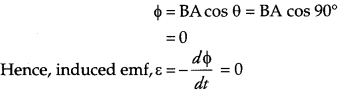Question 3.
At a place, the horizontal component of earth’s magnetic field is B and angle of dip is 60°. What is the value of horizontal component of the earth’s magnetic field at equator ?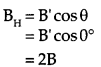Question 4.
Name the junction diode whose I-V characteristics are drawn below.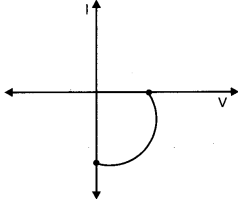This is the characteristic of solar cell.

Question 5.
How is the speed of em-waves in vacuum determined by the electric and magnetic fields? 
The speed of em waves are determined by the ratio of the peak values of electric and magnetic field vectors.
$$c=\frac{E_{0}}{B_{0}}$$

Section-B

Question 6.
How does Ampere-Maxwell law explain the flow of current through a capacitor when it is being charged by a battery ? Write the expression for the displacement current in terms of the rate of change of electric flux. 
During the charging of capacitor, (electric flux between the plates of capacitor keeps on changing, due to which displacement current between the plates is produced. Hence circuit becomes complete and current flow through the circuit.
$$\mathrm{I}_{\mathrm{D}}=\epsilon_{0} \frac{d \phi_{\mathrm{E}}}{d t}$$

Question 7.
Define the distance of closest approach. An a-particle of kinetic energy ‘K’ is bombarded on a thin gold foil. The distance of the closest approach is ‘r’. What will be the distance of closest approach for an a-particle of double the kinetic energy ? 
OR
Write two important limitations of Rutherford nuclear model of the atom.
It is defined as the minimum distance of the charged particle from the nucleus at which initial kinetic energy of the particle is equal to the potential energy due to the charged nucleus.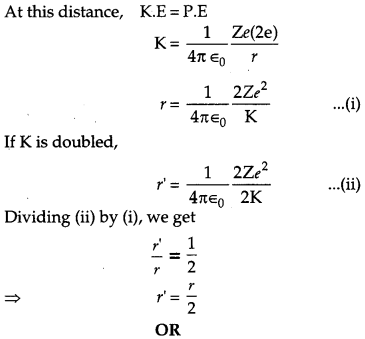Limitations :
(a) It is not in accordance with the Maxwell’s theory and could not explain the stability of an atom.
(b) It did not say anything about the arrangement of electrons in an atom.

Question 8.
Find out the wavelength of the electron orbiting in the ground state of hydrogen atom. 
Answer: We know that, according to de Broglie relation,Question 9.
Define the magnifying power of a compound microscope when the final image is formed at infinity. Why must both the objective and the eyepiece of a compound microscope has short focal lengths ? Explain. 
Magnifying Power : The magnifying power of a compound microscope is defined as the ratio of the angle subtended at the eye by the final image to the angle subtended at the eye by the object, when both the final image and the object are situated at the least distance of distinct vision from the eye. Magnifying power of the compound microscope when the final image is at infinity,
$$m=-\frac{\mathrm{L}}{f_{0}} \times \frac{\mathrm{D}}{f_{e}}$$
From the above equation, we can see that to achieve a large magnification, the objective and eyepiece should have small focal lengths.

Question 10.
Which basic mode of communication is used in satellite communication ? What type of wave propagation is used in this mode ? Write, giving reason, the frequency range used in this mode of propagation. 

Section – C

Question 11.
(a) Find the value of the phase difference between the current and the voltage in the series LCR circuit shown below. Which one leads in phase current or voltage ?
(b) Without making any other change, find the value of the additional capacitor C1, to be connected in parallel with capacitor C, in order to make the power factor of the circuit unity.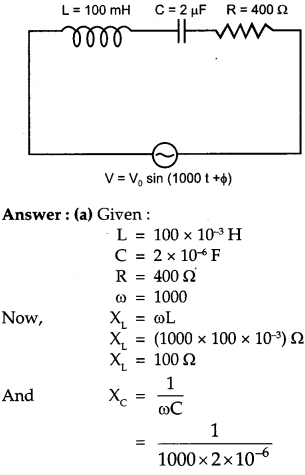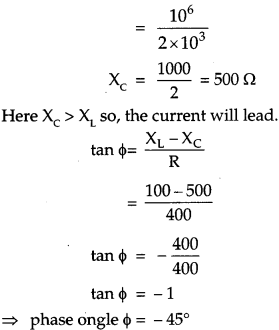Question 12.
Write the two processes that take place in the formation of a p-n junction. Explain with the help of a diagram, the formation of depletion region and barrier potential in a p-n junction. 
Two important processes involved during the formation of p-n junction are diffusion and drift. Electron diffusionFormation of depletion region and barrier potential: At the instant of p-n junction formation, the free electrons near the junction diffuse across the junction into the p region and combines with holes. Thus, on combining with the hole, it makes a negative ion and leaves a positive ion on n-side. These two layers of immobile positive and immobile negative charges form the depletion region.

Further, as electrons diffuse across the junction a point is reached where the negative charge repels any further diffusion of electron. This depletion region now acts as a barrier. Now the external energy is supplied to get the electrons to move across the barrier of electric field. The potential difference required to move the electrons through the electric field is called barrier potential.

Question 13.
(a) Obtain the expression for the cyclotron frequency.
(b) A deuteron and a proton are accelerated by the cyclotron. Can both be accelerated with the same oscillator frequency ? Give reason to justify your answer. 
(a) Suppose the positive ion with charge q moves in a dee with a velocity v, then
\begin{aligned} \frac{m v^{2}}{r} &=\mathrm{qvB} \\ r &=\frac{m v}{q \mathrm{B}} \end{aligned}
where m is the mass and r is the radius of the path of ion in the dee and B is the strength of the magnetic field. The time taken by the ion,
$$\begin{array}{l}{\mathrm{T}=\frac{2 \pi r}{v}} \\ {\frac{1}{f}=\frac{2 \pi r}{v}} \\ {f=\frac{\mathrm{v}}{2 \pi r}} \\ {f=\frac{v}{2 \pi \times \mathrm{mv}}[\mathrm{using}(\mathrm{I})]}\end{array}$$

This frequency is called the cyclotron frequency,
(b) Deuteron and proton have different masses and cyclotron on frequency depends inversly on mass. Hence, cannot be accelerated with the same oscillator frequency.

Question 14.
(a) How does one explain the emission of electrons from a photosensitive surface with the help of Einstein’s photoelectric equation?
(b) The work function of the following metals is given: Na = 2.75 eV, K = 23 eV, Mo = 4.17 eV and Ni = 5.15 eV. Which of these metals will not cause photoelectric emission for radiation of wavelength 3300 A from a laser source placed 1 m away from these metals ? What happens if the laser source is brought nearer and placed 50 cm away ?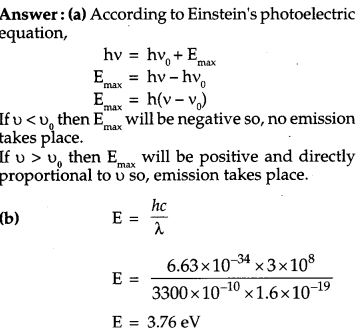The work function of Na and K is less than the energy of incident radiation. ,Therefore, Na and K will cause photoelectric emission while Mo and Ni will not cause photoelectric emission. There will be no effect on photoelectric emission if the source is brought nearer.

Question 15.
A resistance of R draws current from a potentiometer. The potentiometer wire, AB, has a total resistance of R0. A voltage V is supplied to the potentiometer. Derive an expression for the voltage across R when the sliding contact is in the middle of potentiometer wire.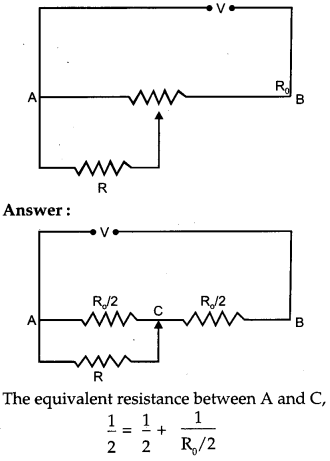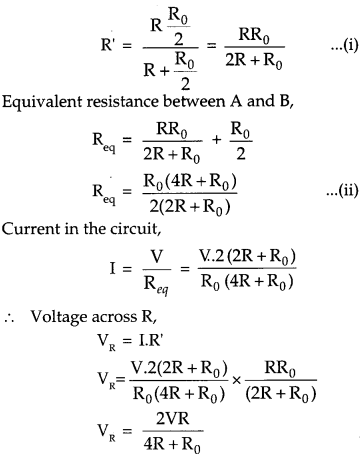Question 16.
Define the term ‘amplitude modulation.’ Explain any two factors which justify the need for modulating a low frequency base-band signal. 

Question 17.
(a) Find equivalent capacitance between A and B in the combination given below. Each capacitor is of 2μF capacitance.(b) If a dc source of 7 V is connected across AB, how much charge is drawn from the source and what is the energy stored in the network? 
(a) In the given figure, C2, C3 and C4 are in parallel so, the equivalent capacitance of parallel capacitors is given by C.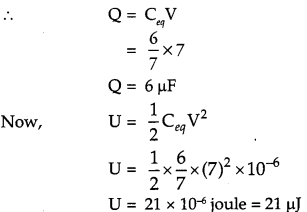Question 18.
(a) Derive the expression for electric field at a point on the equatorial line of an electric dipole.
(b) Depict the orientation of the dipole in
(i) stable,
(ii) unstable equilibrium in a uniform electric field. 
(a) Consider a point P on broad side position of dipole formed of charges +q and -q at separation 2l. The distance of point P from mid point (O) of electric dipole is r. Let $$\overrightarrow{\mathrm{E}_{1}}$$ and $$\overrightarrow{\mathrm{E}_{1}}$$ be the electric field strengths due to charges + q and – q of electric dipole.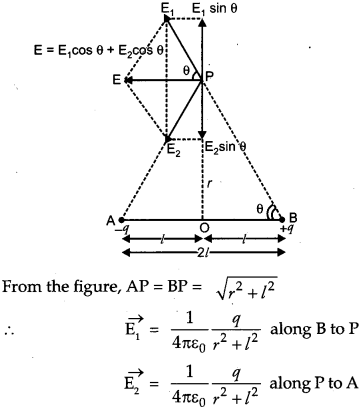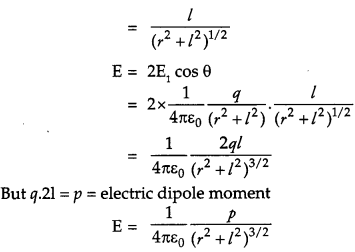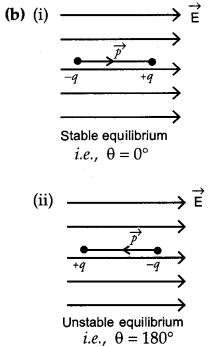Question 19.
(a) A radioactive nucleus ‘A’ undergoes a series of decays as given below: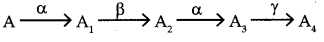The mass number and atomic number of A2 are 176 and 71 respectively. Determine the mass and atomic numbers of A4 and A.
(b) Write the basic nuclear process underlying β+ and β decays.
(a)Question 20.
(a) A ray of light incident on face AB of an equilateral glass prism, shows minimum deviation of 30°. Calculate the speed of light through the prism.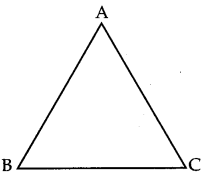(b) Find the angle of incidence at face AB so that the emergent ray grazes along the face AC.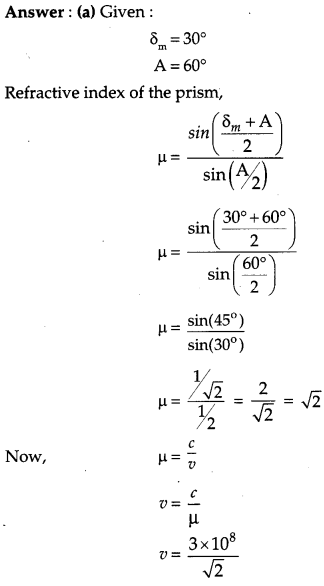Question 21.
For a CE-transistor amplifier, the audio signal voltage across the collector resistance of 2 kΩ is 2 V. Given the current amplification factor of the transistor is 100, find the input signal voltage and base current, if the base resistance is 1 kΩ. 

Question 22.
Describe the working principle of a moving coil galvanometer. Why is it necessary to use
(i) a radial magnetic field and
(ii) a cylindrical soft iron core in a galvanometer? Write the expression for current sensitivity of the galvanometer. Can a galvanometer as such be used for measuring the current ? Explain. 
OR
(a) Define the term ‘self-inductance’ and write its S.I. unit.
(b) Obtain the expression for the mutual inductance of two long co-axial solenoids S1 and S2 wound one over the other, each of length L and radii r1 and r2 and n1 and n, number of turns per unit length, when a current I is set up in the outer solenoid S2.
Moving coil galvanometer
Principle : It works on the principle that a current carrying coil placed in a magnetic field experiences a torque.
Radial magnetic field: It is necessary to eliminate the effect of 0 on torque and hence enable us to make the scale of galvanometer linear.
Cylindrical soft iron core: It makes the magnetic lines of force pointing along the radii of the circle and also due to high permeability it intensifies the magnetic field and hence increases the sensitivity of the galvanometer.
Current sensitivity:
$$I_{s}=\frac{N B A}{K} \text { or } \frac{Q}{I}$$

where Q is the deflection of coil No, it can not be used to measure electric current because it is a highly current sensitive device which would show large deflection even with the passage of small current. And the galvanometer coil is likely to damaged by currents in (mA/A) rang.
OR
(a) Self-inductance: The self inductance of a coil may be defined as the induced emf set up in the coil due to a unit rate of change of current through it.
Its S.I. unit is Henry (H).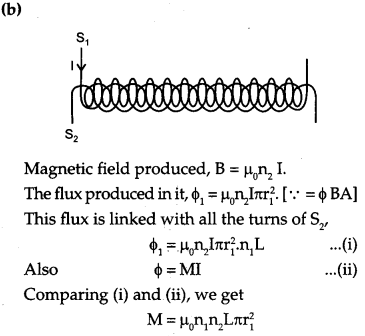Section-D

Question 23.
Mrs. Rashmi Singh broke her reading glasses. When she went to the shopkeeper to order new spects, he suggested that she should get spectacles with plastic lenses instead of glass lenses. On getting the new spectacles, she found that the new ones were thicker than the earlier ones, she asked this question to the shopkeeper but he could not offer satisfactory explanation for this. At home, Mrs. Singh raised the same question to her daughter Anuja who explained why plastic lenses were thicker. 
(a) Write two qualities displayed each by Anuja and her mother.
(b) How do you explain this fact using lens maker’s formula ?
(b) According to lens maker’s formula,
$$\frac{1}{f}=(n-1)\left(\frac{1}{\mathbf{R}_{1}}-\frac{1}{\mathbf{R}_{2}}\right)$$

where f is the focal length, n is the refractive index and R1 and R2 is the radius of curvature of the lens.
Since , ng > np
where, g stands for glass and p stands for plastic. Therefore, we get (ng -1) > (np -1)
Now, using the lens maker’s formula, we see that focal length is inversely proportional to (μ -1).
Hence, fp > fg

Thus, in the case of plastic lens the thickness of the lens should be increased to keep the same focal length as that of the glass lens to give the same power.

Section-E

Question 24.
(a) Draw a labelled diagram of AC generator. Derive the expression for the instantaneous value of the emf induced in the coil.
(b)A circular coil of cross-sectional area 200 cm2 and 20 turns is rotated about the vertical diameter with angular speed of 50 rad s-1 in a uniform magnetic field of magnitude 3.0 x 10-2 T. Calculate the maximum value of the current in the coil. 
OR
(a) Draw a labelled diagram of a step-up transformer. Obtain the ratio of secondary to primary voltage in terms of number of turns and currents in the two coils.
(b) A power transmission line feeds input power at 2200 V to a step-down transformer with its primary winding’s having 3000 turns. Find the number of turns in the secondary to get the power output at 220 V.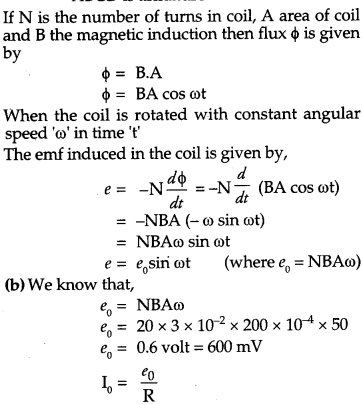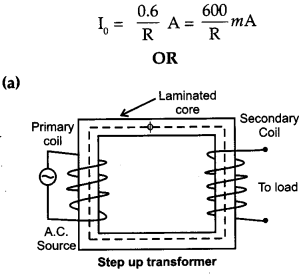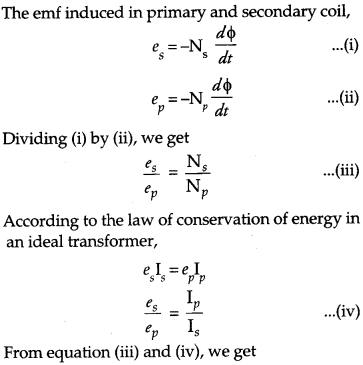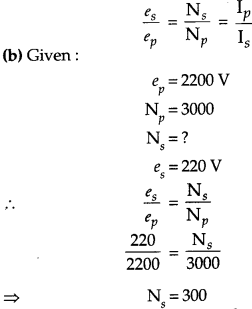Question 25.
(a) Distinguish between unpolarised light and linearly polarised light. How does one get linearly polarised light with the help of a Polaroid ?
(b)A narrow beam of unpolarised light of intensity I0 is incident on a polaroid P1 The light transmitted by it is then incident on a second polaroid P1 with its pass axis making angle of 60° relative to the pass axis of P1 Find the intenstity of the light transmitted by P2. 
OR
(a) Explain two features to distinguish between the interference pattern in Young’s double slit experiment with the diffraction pattern obtained due to a single slit.
(b) A monochromatic light of wavelength 500 nm is incident normally on a single slit of width 0.2 mm to produce a diffraction pattern. Find the angular width of the central maximum obtained on the screen. Estimate the number of fringes obtained in Young’s double slit experiment with fringe width 0.5 mm, which can be accommodated with the region of total angular spread of the central maximum due to single slit. 
(a) Unpolarised light : If the electric component of light vibrates in all the direction in the plane perpendicular to the direction of propagation, the light is known as unpolarised light.
Linearly polarised light: If the vibration of electric components of light are restricted in one direction only in the plane perpendicular to the propagation of light then light is known as linearly polarised light.

A polaroid consists of long chain molecules aligned in a particular direction. The electric vectors along the direction of the aligned molecules get absorbed. So, when an unpolarised light falls on a polaroid, it lets only those of its electric vectors that are oscillating along a direction perpendicular to its aligned molecules to pass through it. The incident light thus gets linearly polarised.
(b)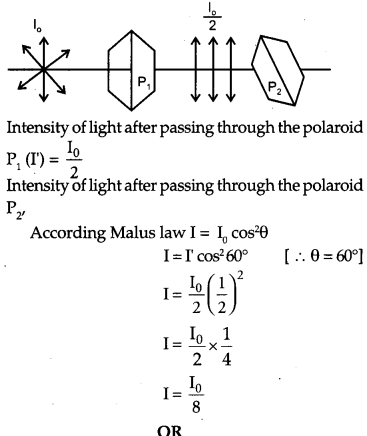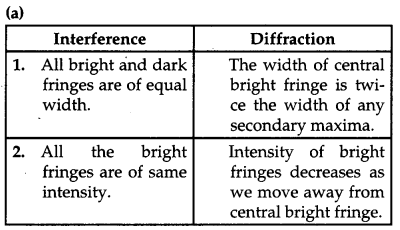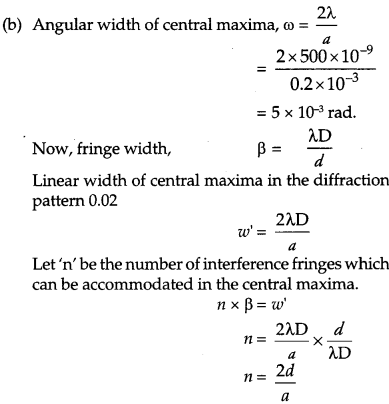Question 26.
(a) Derive an expression for drift velocity of electrons in a conductor. Hence deduce Ohm’s law.
(b) A wire whose cross-sectional area is increasing linearly from its one end to the other, is connected across a battery of V volts. Which of the following quantities remain constant in the wire ?
(i) drift speed
(ii) current density
(iii) electric current
OR
(a) State the two Kirchhoff’s laws. Explain briefly how these rules are justified.
(b) The current is drawn from a cell of emf E and internal resistance r connected to the network of resistors each of resistance r as shown in the figure. Obtain the expression for
(i) the current drawn from the cell and
(ii) the power consumed in the network.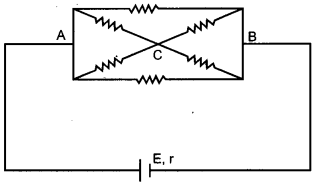(a) Expression of Drift velocity : It is defined as the average velocity gained by the free electrons of a conductor in the opposite direction of the externally applied electric field. If an electron have initial velocity u1 and accelerated for time t1 then velocity attain by it will be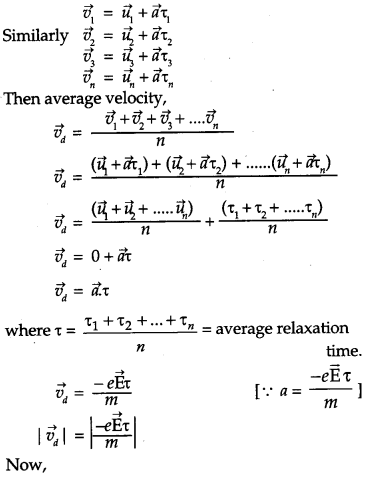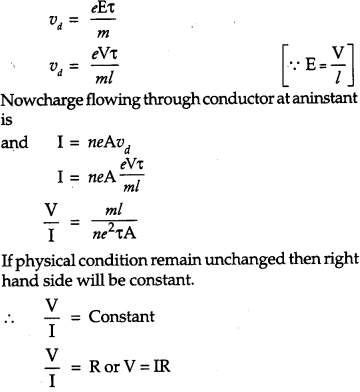Hence  Ohm’s law  is derived.
(b) The electric current will remain constant in the wire as it does not depend upon the cross-sectional area whereas the drift speed, current density, electric field depends upon the increasing area of cross-section with the following relations:OR
(a) Kirchhoff’s laws:
1. Kirchhoff’s first law or junction rule: In an electric circuit, the algebraic sum of currents at any junction is zero.
ΣI= 0
2. Kirchhoff’s second law or loop rule :
Around any closed loop of a network, the algebraic sum of changes in the potential must be zero.
ΣΔV = 0
Justification :
First law is based on the law of conservation of charge and second law is based on the law of conservation of energy.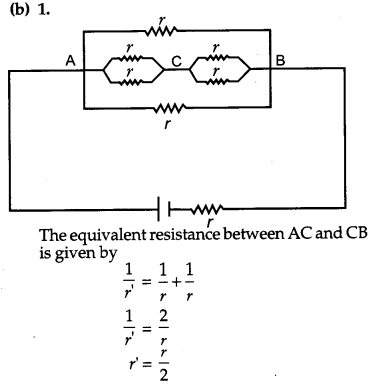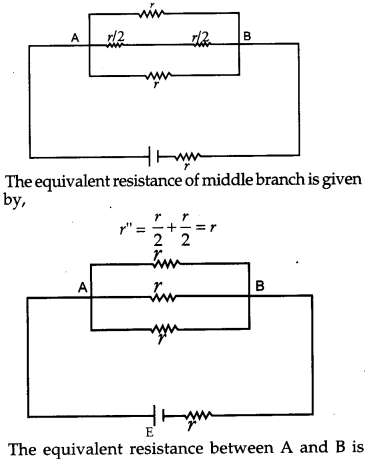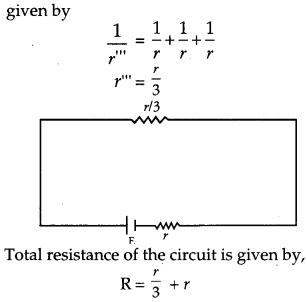### CBSE Previous Year Question Papers Class 12 Physics 2017 Delhi Set-II

Note : Except for the following questions, all the remaining questions have been asked in previous set.

Question 1.
Find the wavelength of the electron orbiting in the first excited state in hydrogen atom.Question 2.
Distinguish between a transducer and a repeater. 

Question 3.
Why should the objective of a telescope have large focal length and large aperture ? Justify your answer.Question 4.
In the study of a photoelectric effect the graph between the stopping potential V and frequency v of the incident radiation on two different metals P and Q is shown below: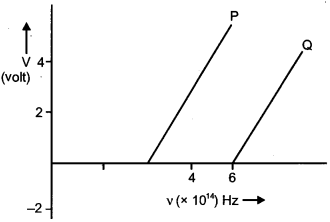Question 5.
(i) Which one of the two metals has higher threshold frequency ?
(ii) Determine the work function of the metal which has greater value.
(iii) Find the maximum kinetic energy of electron emitted by light of frequency 8 × 10-14 Hz for this metal.
(i) Q has higher threshold frequency.
(ii) Work function of Q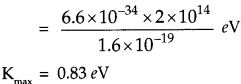Question 6.
A 12 pF capacitor is connected to a 50 V battery. How much electrostatic energy is stored in the capacitor ? If another capacitor of 6 pF is connected in series with it with the same battery connected across the combination, find the charge stored and potential difference across each capacitor. 
Energy stored in the capacitor of capacitance 12 pF,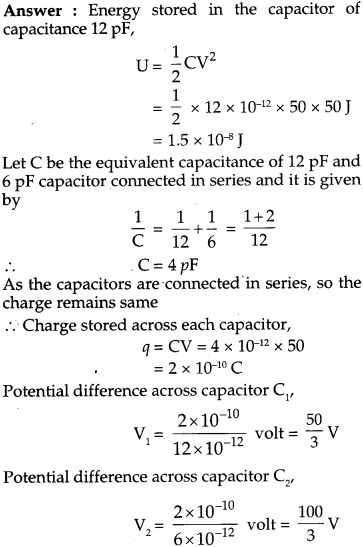Question 7.
A zener diode is fabricated by heavily doping both p and n- sides of the junction. Explain, why ? Briefly explain the use of zener diode as a dc voltage regulator with the help of a circuit diagram. 
By heavily doping both p and n sides of the junction, depletion region formed is very thin, i.e. < 10-6 m. Hence, electric field across the junction is very high (~5 x 106 V/m) even for a small reverse bias voltage. This can lead to a break down during reverse biasing.If the input voltage increases/decreases then current through resistor Rs and Zener diode also increases/decreases. This increases/decreases the voltage drop across Rs without any change in voltage across the zener diode. This is because in the breakdown region, zener voltage remains constant even though the current through the zener diode changes.

Question 8.
A electron of mass me revolves around a nucleuse of charge +Ze. Show that it behaves like a tiny magnetic dipole. Hence prove that the magnetic moment associated with it is expressed as $$\vec{\mu}=-\frac{e}{2 m_{0}} \overrightarrow{\mathrm{L}}$$ where $$\overrightarrow{\mathrm{L}}$$ is the orbital angular momentum of the electron. Give the significance of negative sign. 
Electron in circular motion around the nucleus, constitutes a current loop which behaves like a magnetic dipole.
Current associated with the revolving electron,The negative sign signifies that the angular momentum of the revolving electron is opposite in direction to the magnetic moment associated with it.

Question 9.
(a) Derive the expression for the electric potential due to an electric dipole at a point on its axial line.
(b) Depict the equipotential surfaces due to an electric dipole. 
(a) Consider an electric dipole having charges -q and +q at separation ‘2a’. The dipole moment of dipole is$$\vec{p}=q(2 \vec{a})$$, directed from -q to +q. The electric potential due to dipole is the algebraic sum of potentials due to charges +q and -q.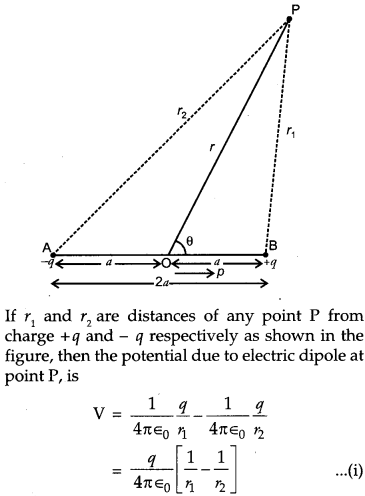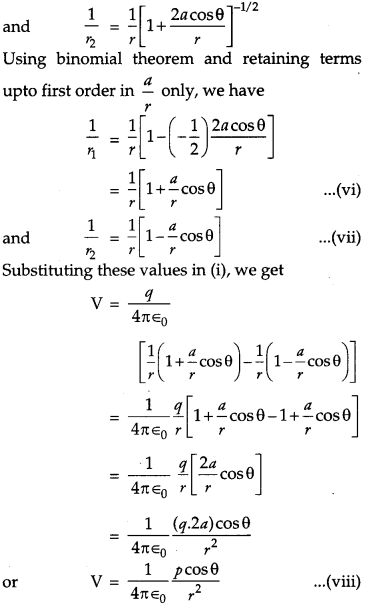### CBSE Previous Year Question Papers Class 12 Physics 2017 Delhi Set-III

Note : Except for the following questions, all the remaining questions have been asked in previous sets.

Question 1.
When are two objects just resolved ? Explain. How can the resolving power of a compound microscope be increased ? Use relevent formula to support your answer. 
When the maxima of diffraction pattern from one object coincide with the minima of second object then they are just resolved.The resolving power of a compound microscope can be increased by increasing µ and by decreasing λ.

Question 2.
(a) What is the line of sight communication ?
(b) Why is it not possible to use sky waves for transmission of T.V. signals ? Up to what distance can a signal be transmitted using an antenna of height ‘h’ ? 

Question 3.
An a-particle and a proton are accelerated through the same potential difference. Find the ratio of their de Broglie wavelengths.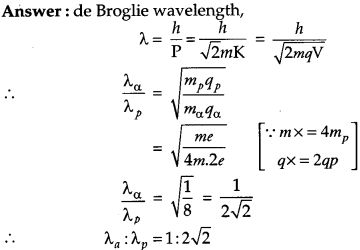Question 4.
(a) State two important features of Einstein’s photoelectric equation.
(b) Radiation of frequency 1015 Hz is incident on two photosensitive surfaces P and Q. There is no photoemission from surface P. Photoemission occurs from surface Q but photoelectrons have zero kinetic energy. Explain these observations and find the value of work function for surface Q.Question 5.
(a) Obtain the expression for the torque $$\vec{\tau}$$ experienced by an electric dipole of dipole moment $$\overrightarrow{\mathrm{p}}$$ in a uniform electric field, $$\overrightarrow{\mathbf{E}}$$ .
(b)What will happen if the field were not uniform ? 
(a)(b) If the field is non-uniform then there will be a net force acting on the dipole. Also, a net torque acting on the dipole which depends on the location of the dipole.

Question 6.
Explain briefly with the help of necessary diagrams, the forward and the reverse biasing of a p-n junction diode. Also draw their characteristic curves in the two cases. 
Forward biasing : If the positive terminal of the battery is connected to the p type semiconductor and negative terminal to the n type semiconductor then it is said to be in forward biased.Reverse Biasing : If the positive terminal of the battery is connected to the n type semiconductor and the negative termal to the p type semiconductor then it is said to be reverse biased.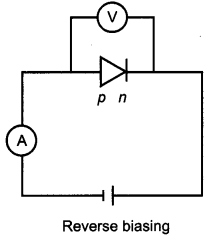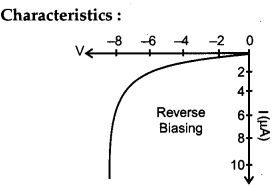Question 7.
Two identical capacitors of 12 pF each are connected in series across a battery of 50 V. How much electrostatic energy is stored in the combination ? If these were connected in parallel across the same battery, how much energy will be stored in the combination now ? Also find the charge drawn from the battery in each case.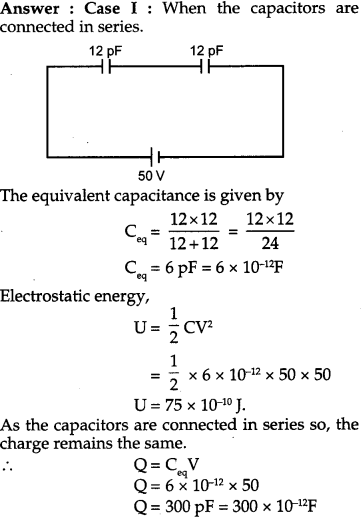Question 8.
(a) Write the expression for the force $$\overrightarrow{\mathbf{F}}$$ acting on a particle of mass m and charge q moving with velocity $$\overrightarrow{\mathbf{v}}$$ in a magnetic field $$\overrightarrow{\mathbf{B}}$$. Under what conditions will it move in
(i) a circular path and
(ii) a helical path ?
(b) Show that the kinetic energy of the particle moving in magnetic field remains constant. 
(a) $$\overrightarrow{\mathrm{F}}=\mathrm{q}(\overrightarrow{\mathrm{v}} \times \overrightarrow{\mathrm{B}})=\mathrm{q} \mathrm{vB} \sin \theta$$